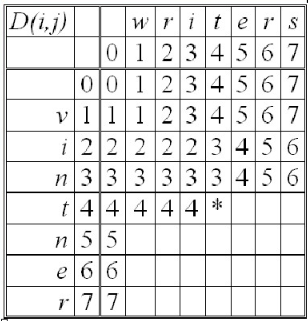# LeetCode 72*. 编辑距离（Python）1. 插入一个字符
2. 删除一个字符
3. 替换一个字符

1. 替换： 在str1变成str2的步骤后，我们将str1a中的a替换为b，就得到str2b (如果a和b相等，就不用操作)
<li>增加： 在str1a变成str2的步骤后，我们再在末尾添加一个b，就得到str2b (str1a先根据已知距离变成str2，再加个b)</li>

<li>删除： 在str1变成str2b的步骤后，对于str1a，我们将末尾的a删去，就得到str2b (str1a将a删去得到str1，而str1到str2b的编辑距离已知)</li>``````class Solution(object):
def minDistance(self, word1, word2):
"""
:type word1: str
:type word2: str
:rtype: int
"""
if word1 is None:
return word2.length
if word2 is None:
return word1.length
dp = [[0 for i in range(len(word2) + 1)] for j in range(len(word1) + 1)]
for i in range(len(word2) + 1):
# dp = 0
dp[i] = i
for j in range(len(word1) + 1):
# dp[j] = 0
dp[j] = j
print(dp)
for i in range(1, len(word1) + 1):
for j in range(1, len(word2) + 1):
if word1[i-1] == word2[j-1]:
dp[i][j] = dp[i - 1][j - 1]
else:
insertion = dp[i][j - 1] + 1
deletion = dp[i - 1][j] + 1
substitution = dp[i - 1][j - 1] + 1
dp[i][j] = min(insertion, deletion, substitution)
return dp[len(word1)][len(word2)]``````

-- 展开阅读全文 --

« 上一篇 07-24